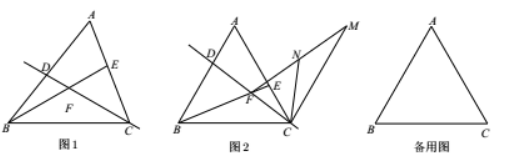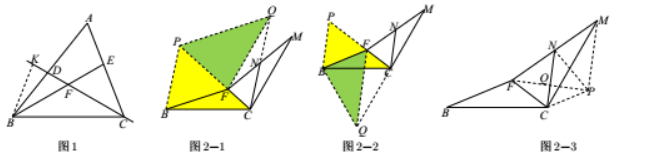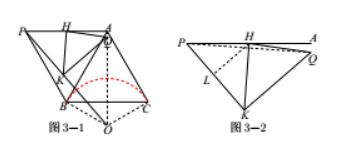(1) 如图 1, 若 $A B > A C$, 且 $B D=C E, \angle B C D=\angle C B E$, 求 $\angle C F E$ 的度数;
(2) 如图 2, 若 $A B=A C$, 且 $B D=A E$, 在平面内将线段 $A C$ 绕点 $C$ 顺时针方向旋转 $60^{\circ}$ 得到线段 $C M$, 连接 $M F$, 点 $N$ 是 $M F$ 的中点, 连接 $C N$. 在点 $D, E$ 远动过程中, 猜想线段 $B F, C F, C N$ 之间存在的数量关系， 并证明你的猜想:
(3) 若 $A B=A C$, 且 $B D=A E$, 将 $\triangle A B C$ 沿直线 $A B$ 翻折至 $\triangle A B C$ 所在平面内得到 $\triangle A B P$, 点 $H$ 是 $A P$ 的中 点,点 $K$ 是线段 $P F$ 上一点, 将 $\triangle P H K$ 沿直线 $H K$ 翻折至 $\triangle P H K$ 所在平面内得到 $\triangle Q H K$, 连接 $P Q$. 在点 $D, E$ 动过程中, 当线段 $P F$ 取得最小值, 且 $Q K \perp P F$ 时, 请直接写出 $\frac{P Q}{B C}$ 的值.(1) 如图 1 , 在射线 $C D$ 上取一点$K$, 使得 $C K=B E, \therefore$
$\triangle C B E \cong \triangle B C K$
\begin{aligned} & \therefore B K=C E=B D, \therefore \\ & \angle B K D=\angle B D K= \\ & \angle C E B=\angle A D F \\ & \therefore \angle A D F+\angle A E F=\angle A E F+\angle C E B=180^{\circ}, \therefore \angle A+\angle D F E=180^{\circ} \\ & \therefore \angle D F E=120^{\circ}, \therefore \angle E F C=60^{\circ} \end{aligned}(2) $\triangle A B E \cong \triangle B C D, \therefore \angle B C F=\angle A B E, \therefore \angle F B C+\angle B C F=60^{\circ}, \therefore \angle B F C=120^{\circ}$

\begin{aligned} & \therefore \angle P B C+\angle P C B=\angle P C B+\angle F C M=120^{\circ}, \therefore \angle P F Q=\angle F C M=\angle P B C \\ & \because P B=P F, \therefore \triangle P F Q \cong \triangle P B C, \therefore \triangle P C Q \text { 为正三角形 } \\ & \therefore B F+C F=P C=Q C=2 C N \end{aligned}

(3)(3) 由 (2) 知 $\angle B F C=120^{\circ}, \therefore F$ 轨迹为红色圆弧. $\therefore P 、 F 、 O$ 三点共线时, $P F$ 取得最小值 此时 $\tan \angle A P K=\frac{A O}{A P}=\frac{2}{\sqrt{3}}, \therefore \angle H P K > 45^{\circ}$
$$\because Q K \perp P F, \therefore \angle P K H=\angle Q K H=45^{\circ} \text {, }$$

$$\therefore \frac{P Q}{B C}=\frac{2+\sqrt{3}}{\sqrt{14}}=\frac{2 \sqrt{14}+\sqrt{42}}{14}$$
①点击 首页查看更多试卷和试题 , 点击查看 本题所在试卷